# Catch up on the latest AI articles# ImageReward: A Reward Model That Learns Human Evaluation In Text-to-image

3 main points
✔️ Propose a reward model ImageReward to predict people's preferences in text-to-image tasks.
✔️ Proposes a method, Reward Feedback Learning (ReFL), which uses the output of ImageReward to directly optimize the image generation model by gradient descent.
✔️ It can generate images that are preferred over existing methods.

written by Jiazheng Xu, Xiao Liu, Yuchen Wu, Yuxuan Tong, Qinkai Li, Ming Ding, Jie Tang, Yuxiao Dong
(Submitted on 12 Apr 2023 (v1), last revised 6 Jun 2023 (this version, v3))

Subjects: Computer Vision and Pattern Recognition (cs.CV); Machine Learning (cs.LG)CODE: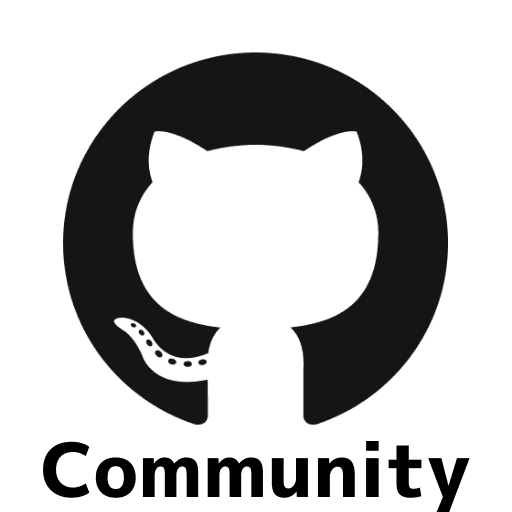The images used in this article are from the paper, the introductory slides, or were created based on them.

## Introduction

The text-to-image model is a model that generates images according to textual instructions, and recent developments have made it possible to generate high-quality images.

However, challenges still remain in the area of alignment, i.e., matching the model's products to human preferences.

Specifically, the following points are noted.

Text-image alignment problem: The number of objects and their relationships specified in the prompt cannot be accurately depicted.

Body problem: The problem of inability to accurately represent human and animal body shapes.

Human aesthetic problem: the problem of portraying people in a way that is outside of normal human tastes.

Toxicity and biases issues: Output of harmful content, etc.

These problems will be difficult to solve simply by improving the architecture of the model or the pre-training data.

In the context of natural language processing, a method called RLHF has been developed for fine-tuning models through reinforcement learning based on feedback data from human evaluations. This allows fine-tuning of language models so that they behave in accordance with human values and preferences. In this method, a module called reward model (RM) is first trained to learn human preferences, which are then used for reinforcement learning. However, it requires the collection of a large amount of human evaluation data, which is labor-intensive and costly in many ways, such as the construction of evaluation methods, the gathering of evaluators, and the validation of evaluations.

To solve these problems, this paper proposes ImageReward, a general-purpose reward model (RM) that learns human preferences in text-to-image. Using this model, we also propose a method called ReFL to optimize the generated model. The example (next figure) shows that it is possible to generate high-quality images that follow the textual instructions as well as human preferences.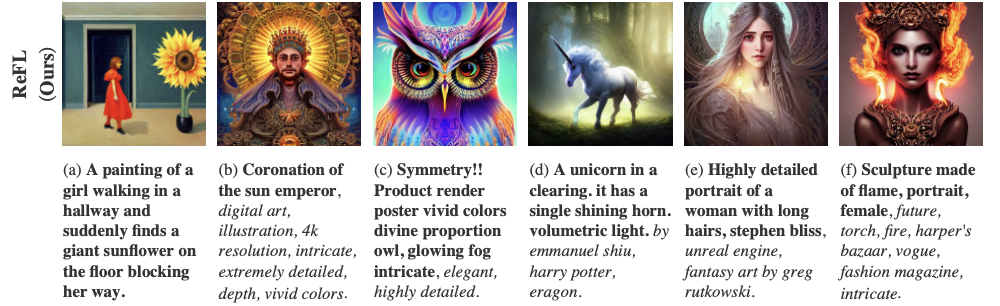In the following, we describe in detail the learning methods and experiments.

## Data Collection

More than 170,000 prompt/image pairs from DiffusionDB, a dataset with 10,000 prompts accompanied by images generated by the Diffusion model, are used as data for evaluation.

The evaluation pipeline begins with a stage in which prompts are categorized and checked for problems, followed by a stage in which scores are evaluated according to alignment (whether the prompts are followed), fidelity (accuracy), and harmlessness (no harm), and finally Finally, the image is ranked according to preference.

The evaluation system is interfaced as shown in the figure below. The data collection resulted in 136892 image prompt pair ratings for 8878 prompts.## Reward model (RM) learning

Following the RM learning style of previous studies, the following loss function is used to learn RM $f_{\theta}$. where the prompt is denoted by $T$ and the generated image is denoted by $x$.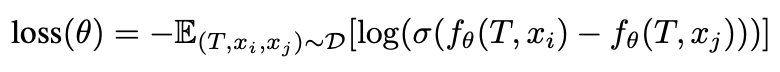Verification has shown that the ImageReward learned in this way can also be used as an evaluation index for text-to-image models. The table below shows how the human ratings correspond to the ImageReward ratings and CLIP ratings when ranking the images generated by the various models. It can be seen that ImageReward's evaluations are consistent with the human evaluations and that they reflect human evaluations better than CLIP's evaluations.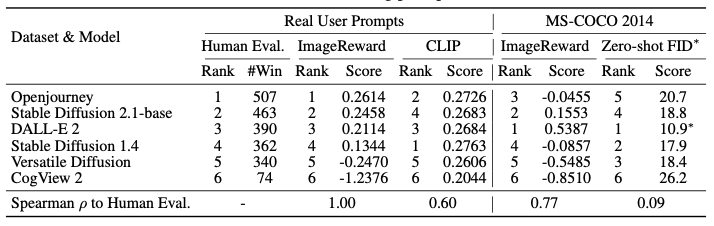## ReFL: fine-tuning of text-to-image models using ImageReward

Consider fine-tuning a text-to-image model using ImageReward.

In the context of natural language processing, reinforcement learning was used to feed RM information back to the language model (RLHF), but diffusion models cannot use the RLHF approach because they are models generated by a multi-step denoising process.

Instead, this paper proposes an algorithm that sets the score output by ImageReward as the objective function and directly performs error back propagation. The former is the objective function of the ImageReward score, and the latter is a regularization term to avoid overlearning and learning instability. where $\theta$ is the parameter of the diffusion model and $g_{\theta}(y_i)$ is the image generated for prompt $y_i$.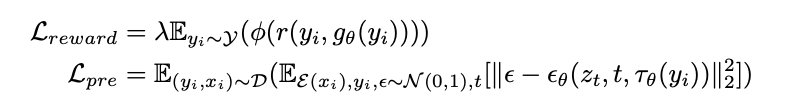## Experiment

The following table shows the accuracy of ImageReward when predicting human preferences: ImageReward shows higher accuracy than other methods (examples using models that calculate the similarity between text and images, such as CLIP and BLIP).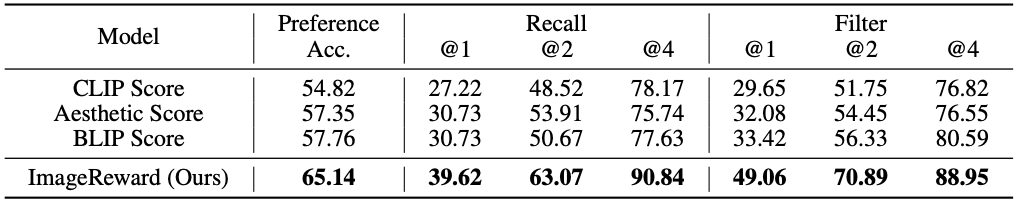### Improvement of Image Generation Performance with ReFL

The following table compares the methods for fine-tuning the Stable Diffusion v1.4 model using ImageReward. It shows the number of times the model was rated higher and its win rate compared to the baseline Stable Diffusion v1.4 model.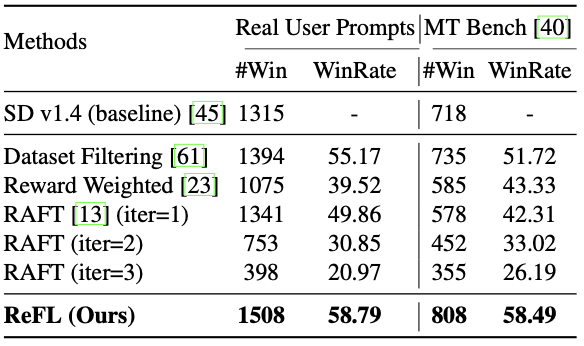The following is a summary of the various previous studies that appear in the table.

Dataset filtering is a technique that uses RM to filter a dataset. The highest and lowest scoring images for a given prompt are selected and used for fine-tuning the generative model.

Reward weighted is a method of fine-tuning the loss function to produce a favorable image by weighting the loss function using RM during fine-tuning of the generative model.

RAFT is a method to improve the performance of the generated model by repeatedly evaluating the generated images and fine-tuning the generated model.

Compared to the above previous studies, the proposed ReFL is found to hit a high level of accuracy.

Unlike previous studies, the proposed ReFL algorithm directly utilizes the reward values from the RM and provides feedback by gradient descent, which is believed to result in a more appreciable image generation.

A qualitative comparison is shown in the following figure.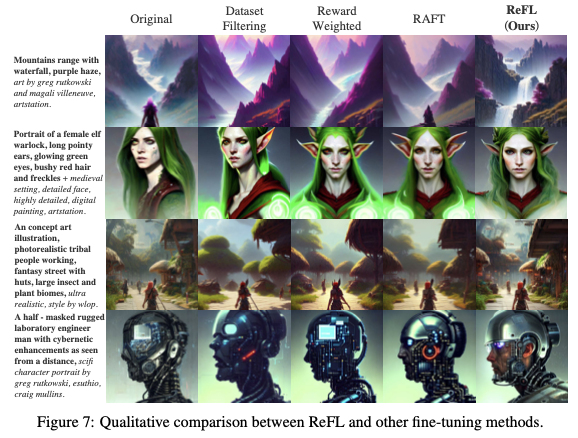## Summary

In the paper introduced in this issue, we proposed ReFL, a method for optimizing image generation models in the field of text-to-image by training a reward model called ImageReward that learns human evaluations and directly using the model by gradient descent, and presented its effectiveness. As shown in this paper, new methods are being proposed for text-to-image datasets, evaluation functions, and applications to generative models, and we will continue to keep an eye on developments in this field.

If you have any suggestions for improvement of the content of the article,